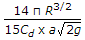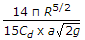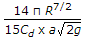# Mechanical Engineering - Hydraulics and Fluid Mechanics

Exercise : Hydraulics and Fluid Mechanics - Section 4
1.
The hydraulic mean depth or the hydraulic radius is the ratio of
area of flow and wetted perimeter
wetted perimeter and diameter of pipe
velocity of flow and area of flow
none of these
Explanation:
No answer description is available. Let's discuss.

2.
The imaginary line drawn in the fluid in such a way that the tangent to any point gives the direction of motion at that point, is known as
path line
stream line
steak line
potential line
Explanation:
No answer description is available. Let's discuss.

3.
A hemispherical tank of radius (R) has an orifice of cross-sectional area (a) at its bottom and is full of liquid. The time required to empty the tank completely isExplanation:
No answer description is available. Let's discuss.

4.
Stoke is the unit of
kinematic viscosity in C. G. S. units
kinematic viscosity in M. K. S. units
dynamic viscosity in M. K. S. units
dynamic viscosity in S. I. units